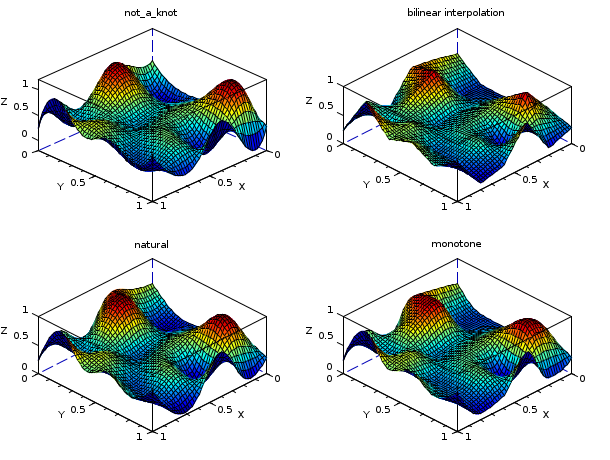Scilab Home page | Wiki | Bug tracker | Forge | Mailing list archives | ATOMS | File exchange
Change language to: Français - Português - 日本語 - Русский

See the recommended documentation of this function

Scilab help >> Interpolation > splin2d

# splin2d

bicubic spline gridded 2d interpolation

### Calling Sequence

`C = splin2d(x, y, z, [,spline_type])`

### Arguments

x,y

strictly increasing row vectors (with at least 2 components) defining the interpolation grid

z

nx x ny matrix (nx being the length of `x` and ny the length of `y`)

spline_type

(optional) a string selecting the kind of bicubic spline to compute

C

a big vector with the coefficients of the bicubic patches (see details in Remarks)

### Description

This function computes a bicubic spline or sub-spline s which interpolates the (xi,yj,zij) points, ie, we have s(xi,yj)=zij for all i=1,..,nx and j=1,..,ny. The resulting spline s is defined by the triplet `(x,y,C)` where `C` is the vector (of length 16(nx-1)(ny-1)) with the coefficients of each of the (nx-1)(ny-1) bicubic patches : on [x(i) x(i+1)]x[y(j) y(j+1)], s is defined by :The evaluation of s at some points must be done by the interp2d function. Several kind of splines may be computed by selecting the appropriate `spline_type` parameter. The method used to compute the bicubic spline (or sub-spline) is the old fashionned one 's, i.e. to compute on each grid point (xi,yj) an approximation of the first derivatives ds/dx(xi,yj) and ds/dy(xi,yj) and of the cross derivative d2s/dxdy(xi,yj). Those derivatives are computed by the mean of 1d spline schemes leading to a C2 function (s is twice continuously differentiable) or by the mean of a local approximation scheme leading to a C1 function only. This scheme is selected with the `spline_type` parameter (see splin for details) :

"not_a_knot"

this is the default case.

"periodic"

to use if the underlying function is periodic : you must have z(1,j) = z(nx,j) for all j in [1,ny] and z(i,1) = z(i,ny) for i in [1,nx] but this is not verified by the interface.

### Remarks

From an accuracy point of view use essentially the not_a_knot type or periodic type if the underlying interpolated function is periodic.

The natural, monotone, fast (or fast_periodic) type may be useful in some cases, for instance to limit oscillations (monotone being the most powerful for that).

To get the coefficients of the bi-cubic patches in a more friendly way you can use `c = hypermat([4,4,nx-1,ny-1],C)` then the coefficient (k,l) of the patch (i,j) (see equation here before) is stored at `c(k,l,i,j)`. Nevertheless the interp2d function wait for the big vector `C` and not for the hypermatrix `c` (note that one can easily retrieve `C` from `c` with `C=c(:)`).

### Examples

```// example 1 : interpolation of cos(x)cos(y)
n = 7;  // a regular grid with n x n interpolation points
// will be used
x = linspace(0,2*%pi,n); y = x;
z = cos(x')*cos(y);
C = splin2d(x, y, z, "periodic");
m = 50; // discretisation parameter of the evaluation grid
xx = linspace(0,2*%pi,m); yy = xx;
[XX,YY] = ndgrid(xx,yy);
zz = interp2d(XX,YY, x, y, C);
emax = max(abs(zz - cos(xx')*cos(yy)));
clf()
plot3d(xx, yy, zz, flag=[2 4 4])
[X,Y] = ndgrid(x,y);
param3d1(X,Y,list(z,-9*ones(1,n)), flag=[0 0])
str = msprintf(" with %d x %d interpolation points. ermax = %g",n,n,emax)
xtitle("spline interpolation of cos(x)cos(y)"+str)``````// example 2 : different interpolation functions on random data
n = 6;
x = linspace(0,1,n); y = x;
z = rand(n,n);
np = 50;
xp = linspace(0,1,np); yp = xp;
[XP, YP] = ndgrid(xp,yp);
ZP1 = interp2d(XP, YP, x, y, splin2d(x, y, z, "not_a_knot"));
ZP2 = linear_interpn(XP, YP, x, y, z);
ZP3 = interp2d(XP, YP, x, y, splin2d(x, y, z, "natural"));
ZP4 = interp2d(XP, YP, x, y, splin2d(x, y, z, "monotone"));
xset("colormap", jetcolormap(64))
clf()
subplot(2,2,1)
plot3d1(xp, yp, ZP1, flag=[2 2 4])
xtitle("not_a_knot")
subplot(2,2,2)
plot3d1(xp, yp, ZP2, flag=[2 2 4])
xtitle("bilinear interpolation")
subplot(2,2,3)
plot3d1(xp, yp, ZP3, flag=[2 2 4])
xtitle("natural")
subplot(2,2,4)
plot3d1(xp, yp, ZP4, flag=[2 2 4])
xtitle("monotone")
show_window()``````// example 3 : not_a_knot spline and monotone sub-spline
//             on a step function
a = 0; b = 1; c = 0.25; d = 0.75;
// create interpolation grid
n = 11;
x = linspace(a,b,n);
ind = find(c <= x & x <= d);
z = zeros(n,n); z(ind,ind) = 1;  // a step inside a square
// create evaluation grid
np = 220;
xp = linspace(a,b, np);
[XP, YP] = ndgrid(xp, xp);
zp1 = interp2d(XP, YP, x, x, splin2d(x,x,z));
zp2 = interp2d(XP, YP, x, x, splin2d(x,x,z,"monotone"));
// plot
clf()
xset("colormap",jetcolormap(128))
subplot(1,2,1)
plot3d1(xp, xp, zp1, flag=[-2 6 4])
xtitle("spline (not_a_knot)")
subplot(1,2,2)
plot3d1(xp, xp, zp2, flag=[-2 6 4])
xtitle("subspline (monotone)")```

• cshep2d — bidimensional cubic shepard (scattered) interpolation
• linear_interpn — n dimensional linear interpolation
• interp2d — bicubic spline (2d) evaluation function

### History

 Version Description 5.4.0 previously, imaginary part of input arguments were implicitly ignored.